Thank you for visiting nature.com. You are using a browser version with limited support for CSS. To obtain the best experience, we recommend you use a more up to date browser (or turn off compatibility mode in Internet Explorer). In the meantime, to ensure continued support, we are displaying the site without styles and JavaScript.

# Dicke-type phase transition in a spin-orbit-coupled Bose–Einstein condensate

## Abstract

Spin-orbit-coupled Bose–Einstein condensates (BECs) provide a powerful tool to investigate interesting gauge field-related phenomena. Here we study the ground state properties of such a system and show that it can be mapped to the well-known Dicke model in quantum optics, which describes the interactions between an ensemble of atoms and an optical field. A central prediction of the Dicke model is a quantum phase transition between a superradiant phase and a normal phase. We detect this transition in a spin-orbit-coupled BEC by measuring various physical quantities across the phase transition. These quantities include the spin polarization, the relative occupation of the nearly degenerate single-particle states, the quantity analogous to the photon field occupation and the period of a collective oscillation (quadrupole mode). The applicability of the Dicke model to spin-orbit-coupled BECs may lead to interesting applications in quantum optics and quantum information science.

## Introduction

Ultracold atomic gases afford unique opportunities to simulate quantum optical and condensed matter phenomena, many of which are difficult to observe in their original contexts1,2. Over the past decade, much theoretical and experimental progress in implementing quantum simulations with atomic gases has been achieved, exploiting the flexibility and tunability of these systems. The recent generation of spin-orbit (SO) coupling in Bose–Einstein condensates (BECs)3,4,5,6 and Fermi gases7,8,9 has brought the simulation of a large class of gauge field-related physics into reach, such as the spin Hall effect10,11,12,13. With such achievements, SO-coupled ultracold atomic gases have emerged as excellent platforms to simulate topological insulators, topological superconductors/superfluids and so on, which have important applications for the design of next-generation spin-based atomtronic devices and for topological quantum computation14,15.

Recently, the ground state properties of a BEC with one-dimensional (1D) or two-dimensional (2D) SO coupling have been analysed theoretically. These investigations have predicted a plane wave or stripe phase for different parameter regimes16,17,18,19,20,21,22,23, agreeing with the experimental observations3. In the plane wave phase of such a SO-coupled BEC, the atomic spins collectively interact with the motional degrees of freedom in the external trapping field, providing a possible analogy to the well-known quantum Dicke model. The Dicke model24, proposed nearly 60 years ago, describes the interaction between an ensemble of two-level atoms and an optical field25. For atom–photon interaction strengths greater than a threshold value, the ensemble of atoms favours to interact with the optical field collectively as a large spin and the system shows an interesting superradiant phase with a macroscopic occupation of photons and non-vanishing spin polarization26,27,28. Even though this model has been solved and is well understood theoretically, the experimental observation was achieved only recently by coupling a BEC to an optical cavity29.

In this work, we experimentally investigate the ground state properties of the plane wave phase of a SO-coupled BEC and show that an insightful analogy to the quantum optical Dicke model can be constructed. The SO coupling in a BEC is realized with a Raman dressing scheme. The system exhibits coupling between momentum states and the collective atomic spin, which is analogous to the coupling between the photon field and the atomic spin in the Dicke model. This analogy is depicted in Fig. 1. By changing the Raman coupling strength, the system can be driven across a quantum phase transition from a spin-polarized phase, marked by a non-zero quasi-momentum, to a spin-balanced phase with zero quasi-momentum, akin to the transition from superradiant to normal phases in Dicke model. Measurements of various physical quantities in these two phases are presented.

## Results

### Theoretical description of the SO-coupled BEC

The Raman dressing scheme is based on coupling two atomic hyperfine states in such a way that a momentum transfer of 2kR in the x direction is accompanied with the change of the hyperfine states, where kR is the photon recoil momentum. The dynamics in the y and z directions are decoupled, which allows us to consider a 1D system in our following discussions (see Methods). The two coupled hyperfine states are regarded as the two orientations of a pseudo-spin 1/2 system. The Raman dressed BEC is governed by the 1D Gross–Pitaevskii (G-P) equation with the Hamiltonian HSO=Hs+HI. Here Hs is the single-particle Hamiltonian and in the basis of the uncoupled states can be written as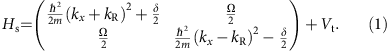Ω is the Raman coupling strength and δ is the detuning of the Raman drive from the level splitting. The recoil energy is defined as ER=2kR2/2m. kx is the quasi-momentum and Vt=x2x2/2 is the external harmonic trap. The many-body interactions between atoms are described by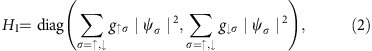where gαβ are the effective 1D interaction parameters (see Methods)30,31. The presence of the interatomic interactions is crucial to observe the Dicke phase transition. For 87Rb atoms, the differences between the spin-dependent nonlinear coefficients are very small and contribute only small modifications to the collective behaviour (see Methods).

The band structure of the non-interacting system with Ω<4ER and δ=0 has two degenerate local minima at quasi-momenta ±q, where q=kR(1−(Ω/4ER)2)1/2. The spin polarization of these two states is finite and opposite to each other. An ensemble of non-interacting atoms occupies both states equally and thus has zero average spin polarization and quasi-momentum. When the nonlinear interactions are taken into account, a superposition state with components located at both degenerate minima generally has an increased energy and is thus not the many-body ground state32. The ground state of the BEC is obtained when the atoms occupy one of the degenerate single-particle ground states (L or R). This is depicted in the inset of Fig. 2a.

The mean field energy associated with a spin-flip in an interacting, harmonically trapped BEC is determined by the coupling between the atomic spin and the many-body ground state harmonic mode. This situation is similar to that of many two-level atoms interacting with a single photon field in an optical cavity24. As shown in the following, the interaction-induced spontaneous symmetry breaking of the ground state allows the mapping of the SO-coupled BEC to the well-known Dicke model, leading to the prediction of an intriguing quantum phase transition when the Raman coupling strength is varied33.

### Mapping to the Dicke model

The realization of the Dicke transition requires two conditions: a single mode that interacts with all atoms and the thermodynamic (large atom number) limit. While the latter is naturally fulfilled in our SO-coupled BECs with N>104 atoms, the first condition is driven by the many-body interaction between atoms. Note that the mean field interaction energy contains two types of terms (see Methods): a term proportional to the overall interaction strength (g↑↑+g↓↓+2g↑↓) that prefers a uniform density (the plane wave phase) and a term proportional to the difference of the interaction strengths in different spin states (g↑↑−g↑↓) that prefers spatial modulation of the density. In the experiment, the first term dominates for a reasonably large Ω and the plane wave phase is preferred (that is, possesses the lowest energy)22. In this phase, all atoms collectively interact with a single plane wave mode, similar to atoms confined in a cavity interacting only with the cavity mode.

To see the connection between the SO-coupled BEC and the Dicke model, the interacting many-body ground state is first expressed in terms of the harmonic trap mode (see Methods). Setting px=kx=i(x/2)1/2(aa), the N-particle Hamiltonian can be written as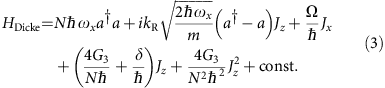where the uniform approximation has been adopted to treat the nonlinear interaction term,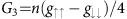, n is the local density. Jx,z are the collective spin operators defined as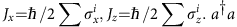is the occupation number of the harmonic trap mode. The differences between the interaction energies contribute an effective detuning term and a nonlinear term in the large spin operator,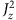. However, these terms are small for the experimental states chosen and thus are ignored in the following analysis. For δ=0, the Hamiltonian of the first line in equation (3) is equivalent to the Dicke model24. A quantum phase transition between the normal phase and a superradiant phase can be driven by changing the Raman coupling strength Ω.

The critical point for the phase transition can be derived using the standard mean field approximation34,35,36 yielding Ωc=4ER (note that theterm yields a small correction −4G3/N to Ωc, which is neglected here). When Ω<Ωc, the Dicke model predicts that the dependence of the order parameter on the Raman coupling strength is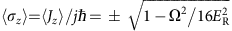. For Ω>Ωc, one obtains 〈Jz〉/j=0 and 〈σx〉=〈Jx〉/j=1 (j=N/2). This scaling is confirmed in our numerical simulation of the G-P equation. The ground state of the BEC is obtained through an imaginary time evolution. The spin polarization can be calculated as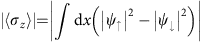. The absolute value of 〈σz〉 is taken since ±q are spontaneously chosen. The scaling of |〈Jz〉|/(j) is shown in Fig. 2 and clearly is consistent with the experimental data described in the following.

### Experimental procedure

To experimentally probe this system, we adiabatically Raman dress a BEC of 87Rb atoms in the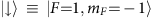and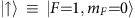hyperfine states (see Methods). A magnetic bias field is applied that generates a sufficiently large quadratic Zeeman splitting such that the |F=1,mF=1〉 state can be neglected. The system can thus be treated as an effective two-state system. To analyse the bare state composition, all lasers are switched off and the atoms are imaged after time-of-flight in a Stern–Gerlach field. This separates the bare states along the vertical axis of the images. The absolute value of the spin polarization, given by |(NN)|/(N+N), and the quasi-momentum are directly measured for condensed atoms at ±q. The experimentally measured absolute value of the spin polarization for various Raman coupling strengths Ω spanning the quantum phase transition is shown in Fig. 2a.

An experimental investigation of the collective choice of the atoms to occupy a single minimum in the dispersion relation is presented in Fig. 3. For this data, the BEC is adiabatically prepared in a Raman dressed state with Ω=3ER and a detuning δ of either ±5.4ER. The detuning is then linearly swept to a final value in 50 ms. The left/right relative occupation of the BEC, defined by (N+qNq)/(N+q+Nq), provides a measure for the relative occupation of the dressed states at the quasi-momentum of the two dispersion minima. The data presented in Fig. 3 exhibits a hysteretic effect dependent on the sign of the initial detuning ±δ. The width of the hysteresis depends on the chosen sweep rate of the detuning (see Methods). While these sweeps are slow enough for the BEC to follow a chosen minimum of the band structure, the transition between the two possible minima is non-adiabatic. Sweeps causing a reversal of the energetic order of the two minima eventually generate heating of the BEC, shown in Fig. 3c. Only values of δ where the BEC is not heated are plotted in Fig. 3a,b. Recently, a similar energy dispersion exhibiting a double well structure was also observed for a BEC in a shaken optical lattice, where the analogous spontaneous occupation of a single band mimimum was also found37. However, such a system lacks the linear coupling between momentum and spin presented in our system (Equation 1), and thus cannot be mapped to the Dicke model.

From the effective Dicke model, we also obtain the quantity analogous to the photon field excitation, as well as the ground state energy of the system. The photon number Np is related to the quasi-momentum q of the SO-coupled BEC: Np=q2 after a constant coefficient is scaled to unity (see Methods). As seen from the experimental measurements and numerical simulations in Fig. 4a, the superradiant phase has a macroscopic photon excitation, while the photon field vanishes in the normal phase. The ground state energy Es of a single particle, as obtained from G-P simulations, is plotted in Fig. 4b and is in agreement with the predictions of the Dicke model. The second-order derivative of Es is discontinuous at the critical point Ω=4ER, reinforcing the nature of the quantum phase transition. The red circles in Fig. 4b show the density-dependent mean field interaction energy in the numerical simulations, contributing ~0.2ER to the total energy.

### Collective excitations

It is well known that various physical quantities may change dramatically across a quantum critical point38. As a particular example, we investigate the quadrupole collective excitation of a SO-coupled BEC. In BECs without SO coupling, the quadrupole excitation frequency only depends on the trapping geometry and on the ratio of the kinetic energy to the trapping energy39. Measurements and G-P simulations of the quadrupole-mode frequencies for SO-coupled BECs are shown in Fig. 5 as a function of the Raman coupling strength. For this data, the quadrupole oscillation period is scaled by the period measured for an off-resonant case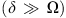. This removes a dependence on the trapping geometry that changes with the Raman coupling strength in the experiment. A peak in the oscillation period around the phase transition is observed. In the G-P simulations, a quadrupole oscillation amplitude of ~0.2kR was used, whereas the oscillation amplitude in the experimental results varied from 0.15kR to 0.55kR. In SO-coupled systems, the hydrodynamic-mode frequencies depend strongly on the oscillation amplitude4. This may contribute to the variation of the experimental data. The numerical results reveal that the oscillations of the BEC are undamped in both spin-balanced and -polarized phases, but show strong damping in the transition region. The behaviour of the quadrupole mode provides an additional signature of the quantum phase transition. Similar experimental behaviour has been observed for the collective dipole motion4.

### Finite detuning

While the analysis above has focused on the case of δ=0, a finite detuning leads to the realization of the generalized Dicke Hamiltonian40. For finite detuning, the sharp quantum phase transition vanishes and the BEC is always in a superradiant (spin-polarized) phase, as shown in Fig. 6. The inclusion of this parameter demonstrates the completeness in the mapping of the SO-coupled BEC to a Dicke-type system.

## Discussion

We have demonstrated that varying the Raman coupling strength can drive a harmonically trapped SO-coupled BEC across a quantum phase transition from a spin-balanced to a spin-polarized ground state. This is a realization of the long-sought phase transition from a normal to a superradiant phase in the quantum Dicke model. The corresponding spin polarization and photon field occupation are calculated for the Dicke model and agree with the experimental measurements and the numerical simulations. The ground state energy is found to be consistent with our numerical simulations as well. Using SO-coupled BECs to study the Dicke model physics may have important applications in quantum information and quantum optics including spin squeezing, quantum entanglement and so on.

## Methods

### Experimental set-up and parameters

For the experiments presented in this article, the BECs are held in a crossed optical dipole trap with frequencies {ωx, ωy, ωz,} within the range of 2π × {12–34,134,178} Hz, respectively. Depending on the Raman coupling strength and crossed dipole trap potential, BECs of 2 × 104 to 10 × 104 atoms are loaded into the dressed state. Small atom numbers are favourable in this trapping geometry to reduce collisions between atoms differing by 2kR during time-of-flight. The Raman beams, intersecting under an angle of π/2, are individually oriented at an angle of π/4 from the long axis of the BEC. They are operated between the D1 and D2 lines of 87Rb in the range of 782.5−790 nm. A 10-G magnetic bias field is applied along the long axis of the BEC and produces a quadratic Zeeman shift of ~7.4ER. The BEC is imaged after performing Stern–Gerlach separation during a 11.5-ms time-of-flight expansion. The images directly reveal the spin and momentum distributions.

For each value of Ω, δ=0 is experimentally identified by finding the Raman laser detuning for which the spin polarization is minimized (normal phase) or for which the BEC switches between the two degenerate states (superradiant phase). This method compensates for the presence of the third atomic hyperfine state (|1, +1〉) in the F=1 manifold. The magnetic bias field was actively stabilized using a technique similar to the one described in ref. 41.

### Experimental preparation methods

To experimentally measure the absolute value of the spin polarization |〈σz〉| and the photon field occupation (shown in Figs 2 and 4), we start with a BEC in the bare state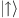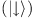and adiabatically turn on the Raman dressing at δ=5.4ER(−5.4ER). The initial state and the dressed state have similar spin compositions. This is followed by a linear sweep of the detuning to a final value in 50–100 ms. An example of results obtained with this loading method is shown in Fig. 7 for the normal phase of the Dicke model with Ω=6ER. This loading method produces BECs with only minimal excitations when the final detuning value is chosen near δ=0. As seen in Fig. 3, this method can cause a hysteresis-like feature at small |δ|.Figure 7: Effect of a small detuning on the normal phase.

An alternative loading method, as opposed to ramps of δ, is investigated by fixing the laser detuning while Ω is increased. The resulting L/R relative occupation measurement is shown in Fig. 8. Here the BEC is loaded to the desired parameters in ~60 ms by only increasing the Raman beam intensities. This is followed by a 150-ms wait time, allowing for excitations to be dissipated. While this wait time is long, there may still be some residual small amplitude collective excitations, such as dipole oscillations. When this technique is used in Fig. 8, the BEC occupies only one of the two minima for all but small detunings. In the range where the BEC fails to load to a single point in the dispersion relation, the quasi-momentum (shown in Fig. 8b, strongly deviates from the single-particle expectation and the BEC acquires excitations. While this loading method does not result in the occupation of the ground state at δ=0, the BEC does choose a single minimum for detunings much smaller than the chemical potential μ, which is on the order of 0.55ER for the data shown. Similar results are obtained by adding sufficient wait time following the linear sweeps of δ performed for Fig. 3. A related effect has been observed in ref. 42.

The quadrupole frequency measurements begin with a BEC in the |F, mF〉=|1, −1〉 state in the crossed dipole trap. The two Raman beam intensities are linearly increased, one after the other, over 30–150 ms. This loading method, performed with the Raman beams at 782.5 nm, generates large quadrupole and small dipole excitations. To simplify the analysis, only the first few oscillations of the quadrupole mode are fit to an undamped oscillation. In the numerical simulations, the quadrupole mode is excited by a sudden jump of the trap frequency along the SO coupling direction. Evolution time is added to observe the temporal oscillation of the condensate width that reveals the quadrupole-mode frequency.

### Dimension reduction from 3D to 1D

The dynamics of SO-coupled BECs and their mapping to the Dicke model are studied on the basis of the 1D G-P equation, although the experimental system is three dimensional (3D) with strong confinements along two directions. The SO coupling is along the elongated direction of the trap, therefore the transverse degrees of freedom do not couple with the internal spin states. Assuming the harmonic ground states along the transverse directions, the effective 1D nonlinear interaction coefficients, g1D, can be approximately obtained from 3D through g1D=g3D/lylz, where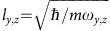are the harmonic characteristic lengths along the transverse directions and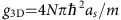is the 3D nonlinear interaction coefficient. For the study of the quadrupole mode, we simulate the 2D G-P equation in the xy plane to compare with the experimental observations, where the dimension reduction gives g2D=g3D/lz.

### Mapping to the Dicke-type Hamiltonian

In the mean field approximation, the single-particle Hamiltonian is given by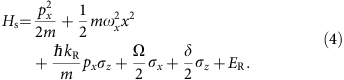As discussed in the main text, the large nonlinear interactions enable the atoms to collectively occupy the same many-body ground state, forming a single mode. For an ensemble of N interacting bosonic atoms, we define the collective spin operators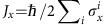and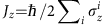. Substituting these collective operators into the N-particle Hamiltonian and using the harmonic mode operator,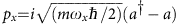, we obtainwhich corresponds to the generalized Dicke model.

The interaction term in the mean field approximation is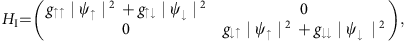which can also be formally expressed using collective operators. We define the variables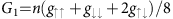,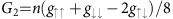and(ref. 22), where n is the average density of the BEC. For the parameters used in our experiment, we have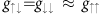, thus G3=2G2≈0 and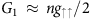.

Because of the normalization condition of the BEC wave function, it is easy to see that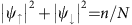and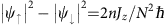(Jz=N/2 when all atoms are in the spin-up state). With these notations, the Hamiltonian can be mapped to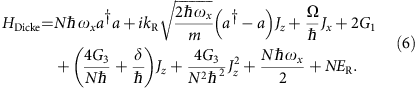Note that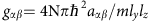is proportional to N, thus all the terms in the above Hamiltonian scale as N.

### Dicke phase transition

The critical point for the phase transition of the Dicke model can be derived using the mean field coherent state36, where the mean field ansatz of the ground state wave function is given by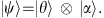Here the spatial coherent state |α〉 is defined by a|α〉=α|a〉, and the spin coherent state |θ〉 is defined as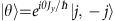with j=N/2 for spin 1/2 atoms and θ [0,2π].

In the absence of the detuning term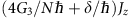, the ground state energy of the Dicke Hamiltonian is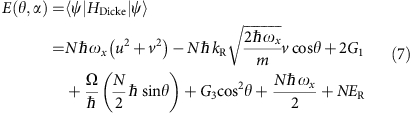where u and v are the real and imaginary parts of α, that is, α=u+iv. Minimizing E(θ,α) with respect to u and v leads to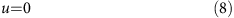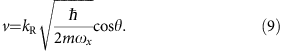Thus the ground state energy becomes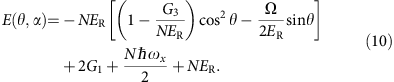Further minimization of E(θ,α) with respect to θ leads to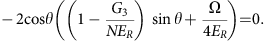Defining Ωc=4ER(1−G3/NER), we obtain two different regions:

1. 1

Ω>Ωc: there is only one solution that minimizes the mean field energy: cosθ=0 and sinθ=−1. In this case, the spin polarization is zero and the corresponding phase is the spin-balanced normal phase.

2. 2

Ω<Ωc: the energy is minimized for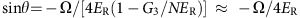and there are two possible values for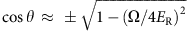, corresponding to a BEC occupying the left or right band minimum.

### Ground state properties in the Dicke model

In the following, we neglect the constant mean field energy terms and the small G3 terms, therefore Ωc≈4ER. In the region Ω>Ωc, the system is in the normal phase and v=u=0. The mean photon number is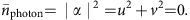The spin polarizations become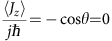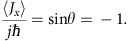The ground state energy per particle isWe denote the first two terms as the single-particle energy Es.

In the region Ω<Ωc, the system is in the superradiant phase with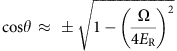and the mean photon number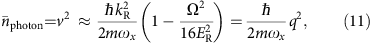where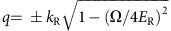is the quasi-momentum of the BEC. Note that the average photon number depends on the trapping frequency and in the main text we rescale it to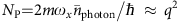. The spin polarization in the superradiant phase is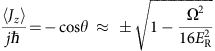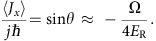Finally, the ground state energy per particle is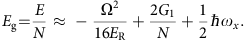We denote the first term as the single-particle energy Es. The energy expressions for Ω<4ER and Ω>4ER are consistent, they are continuous at Ω=4ER.

How to cite this article: Hamner, C. et al. Dicke-type phase transition in a spin-orbit coupled Bose-Einstein condensate. Nat. Commun. 5:4023 doi: 10.1038/ncomms5023 (2014).

## References

1. 1

Bloch, I., Dalibard, J. & Nascimbène., S. Quantum simulations with ultracold quantum gases. Nat. Phys. 8, 267–276 (2012).

2. 2

Bloch, I., Dalibard, J. & Zwerger., W. Many-body physics with ultracold gases. Rev. Mod. Phys. 80, 885–964 (2008).

3. 3

Lin, Y.-J., Garcia, K. J. & Spielman, I. B. Spin-orbit-coupled Bose-Einstein condensates. Nature 471, 83–86 (2011).

4. 4

Zhang, J.-Y. et al. Collective dipole oscillation of a spin-orbit coupled Bose-Einstein condensate. Phys. Rev. Lett. 109, 115301 (2012).

5. 5

Qu, C., Hamner, C., Gong, M., Zhang, C. & Engels, P. Observation of Zitterbewegung in a spin-orbit coupled Bose-Einstein condensate. Phys. Rev. A 88, 021604(R) (2013).

6. 6

Olson, A. J. et al. Tunable Landau-Zener transitions in a spin-orbit coupled Bose-Einstein condensate, Preprint at http://arXiv.org/abs/1310.1818 (2013).

7. 7

Wang, P. et al. Spin-orbit coupled degenerate Fermi gases. Phys. Rev. Lett. 109, 095301 (2012).

8. 8

Cheuk, L. W. et al. Spin-injection spectroscopy of a spin-orbit coupled Fermi gas. Phys. Rev. Lett. 109, 095302 (2012).

9. 9

Williams, R. A., Beeler, M. C., LeBlanc, L. J., Jimenez-Garcia, K. & Spielman, I. B. A Raman-induced Feshbach resonance in an effectively single-component Fermi gas. Phys. Rev. Lett. 111, 095301 (2013).

10. 10

Aidelsburger, M. et al. Realization of the Hofstadter Hamiltonian with ultracold atoms in optical lattices. Phys. Rev. Lett. 111, 185301 (2013).

11. 11

Miyake, H., Siviloglou, G. A., Kennedy, C. J., Burton, W. C. & Ketterle, W. Realizing the Harper Hamiltonian with laser-assisted tunneling in optical lattices. Phys. Rev. Lett. 111, 185302 (2013).

12. 12

Beeler, M. C. et al. The spin Hall effect in a quantum gas. Nature 498, 201–204 (2013).

13. 13

Galitski, V. & Spielman, I. B. Spin-orbit coupling in quantum gases. Nature 494, 49–54 (2013).

14. 14

Hasan, M. Z. & Kane, C. L. Colloquium: topological insulators. Rev. Mod. Phys. 82, 3045–3067 (2010).

15. 15

Qi, X.-L. & Zhang, S.-C. Topological insulators and superconductors. Rev. Mod. Phys. 83, 1057–1110 (2011).

16. 16

Wang, C., Gao, C., Jian, C.-M. & Zhai, H. Spin-orbit coupled spinor Bose-Einstein condensates. Phys. Rev. Lett. 105, 160403 (2010).

17. 17

Wu, C.-J., Mondragon-Shem, I. & Zhou, X.-F. Unconventional Bose-Einstein condensations from spin-orbit coupling. Chin. Phys. Lett. 28, 097102 (2011).

18. 18

Ho, T.-L. & Zhang, S. Bose-Einstein condensates with spin-orbit interaction. Phys. Rev. Lett. 107, 150403 (2011).

19. 19

Sinha, S., Nath, R. & Santos, L. Trapped two-dimensional condensates with synthetic spin-orbit coupling. Phys. Rev. Lett. 107, 270401 (2011).

20. 20

Zhang, Y., Mao, L. & Zhang, C. Mean-field dynamics of spin-orbit coupled Bose-Einstein condensates. Phys. Rev. Lett. 108, 035302 (2012).

21. 21

Hu, H., Ramachandhran, B., Pu, H. & Liu, X.-J. Spin-orbit coupled weakly interacting Bose-Einstein condensates in harmonic traps. Phys. Rev. Lett. 108, 010402 (2012).

22. 22

Li, Y., Pitaevskii, L. P. & Stringari, S. Quantum tricriticality and phase transitions in spin-orbit coupled Bose-Einstein condensates. Phys. Rev. Lett. 108, 225301 (2012).

23. 23

Zhou, X., Li, Y., Cai, Z. & Wu, C. Unconventional states of bosons with the synthetic spin-orbit coupling. J. Phys. B: At. Mol. Opt. Phys. 46, 134001 (2013).

24. 24

Dicke, R. H. Coherence in spontaneous radiation processes. Phys. Rev. 93, 99–110 (1954).

25. 25

Ritsch, H., Domokos, P., Brennecke, F. & Esslinger, T. Cold atoms in cavity-generated dynamical optical potentials. Rev. Mod. Phys. 85, 553–601 (2013).

26. 26

Hepp, K. & Lieb, E. H. On the superradiant phase transition for molecules in a quantized radiation field: the Dicke maser model. Ann. Phys. 76, 360 (1973).

27. 27

Wang, Y. K. & Hioe, F. T. Phase transition in the Dicke model of superradiance. Phys. Rev. A 7, 831 (1973).

28. 28

Gross, M. & Haroche, S. Superradiance: an essay on the theory of collective spontaneous emission. Phys. Rep. 93, 301–396 (1982).

29. 29

Baumann, K., Guerlin, C., Brennecke, F. & Esslinger, T. Dicke quantum phase transition with a superfluid gas in an optical cavity. Nature 464, 1301–1306 (2010).

30. 30

Ho, T.-L. Spinor Bose condensates in optical traps. Phys. Rev. Lett. 81, 742–745 (1998).

31. 31

Widera, A. et al. Precision measurement of spin-dependent interaction strengths for spin-1 and spin-2 87Rb atoms. New J. Phys. 8, 152 (2006).

32. 32

Higbige, J. & Stamper-Kurn, D. M. Generating macroscopic quantum-superposition states in momentum and internal-state space from Bose-Einstein condensates with repulsive interactions. Phys. Rev. A 69, 053605 (2004).

33. 33

Zhang, Y., Chen, G. & Zhang, C. Tunable spin-orbit coupling and quantum phase transition in a trapped Bose-Einstein condensate. Sci. Rep. 3, 1937 (2013).

34. 34

Emary, C. & Brandes, T. Quantum chaos triggered by precursors of a quantum phase transition: the Dicke model. Phys. Rev. Lett. 90, 044101 (2003).

35. 35

Emary, C. & Brandes, T. Chaos and the quantum phase transition in the Dicke model. Phys. Rev. E 67, 066203 (2003).

36. 36

Bakemeier, L., Alvermann, A. & Fehske, H. Quantum phase transition in the Dicke model with critical and noncritical entanglement. Phys. Rev. A 85, 043821 (2012).

37. 37

Parker, C. V., Ha, L.-C. & Chin, C. Direct observation of effective ferromagnetic domains of cold atoms in a shaken optical lattice. Nat. Phys. 9, 769–774 (2013).

38. 38

Sachdev, S. Quantum Phase Transitions Cambridge University Press (1999).

39. 39

Stringari, S. Collective excitations of a trapped Bose-condensed gas. Phys. Rev. Lett. 77, 2360–2363 (1996).

40. 40

Emary, C. & Brandes, T. Phase transitions in generalized spin-boson (Dicke) models. Phys. Rev. A 69, 053804 (2004).

41. 41

Dedman, D. J., Dall, R. G., Byron, L. J. & Truscott, A. G. Active cancellation of stray magnetic fields in a Bose-Einstein condensation experiment. Rev. Sci. Instrum. 78, 024703 (2007).

42. 42

Ji, S.-C. et al. Experimental determination of the finite-temperature phase diagram of a spin-orbit coupled Bose gas. Nat. Phys. 10, 314–320 (2014).

## Acknowledgements

C.H., J.C. and P.E. acknowledge funding from NSF and ARO. C.Q., Y.Z., M.G. and C.Z. are supported by ARO (W911NF-12-1-0334), DARPA-YFA (N66001-10-1-4025), AFOSR (FA9550-13-1-0045) and NSF-PHY (1249293). M.G. is supported by Hong Kong RGC/GRF Projects (Nos. 401011 and 2130352) and the Chinese University of Hong Kong (CUHK) Focused Investments Scheme.

## Author information

Authors

### Contributions

C.H., Y.Z., C.Z. and P.E. conceived the experiment and theoretical modelling; C.H., J.C. and P.E. performed the experiments; C.Q., Y.Z., M.G. performed the theoretical calculations; C.Z. and P.E. supervised the project.

### Corresponding authors

Correspondence to Chuanwei Zhang or Peter Engels.

## Ethics declarations

### Competing interests

The authors declare no competing financial interests.

## Rights and permissions

Reprints and Permissions

• ### Spontaneous symmetry breaking in persistent currents of spinor polaritons

• Evgeny Sedov
• Sergey Arakelian
• Alexey Kavokin

Scientific Reports (2021)

• ### Spin current generation and relaxation in a quenched spin-orbit-coupled Bose-Einstein condensate

• Chuan-Hsun Li
• Chunlei Qu
• Yong P. Chen

Nature Communications (2019)

• ### Phase-factor-dependent symmetries and quantum phases in a three-level cavity QED system

• Jingtao Fan
• Lixian Yu
• Suotang Jia

Scientific Reports (2016)

• ### Spin-momentum coupled Bose-Einstein condensates with lattice band pseudospins

• M. A. Khamehchi
• Chunlei Qu
• P. Engels

Nature Communications (2016)

• ### Qubit lattice coherence induced by electromagnetic pulses in superconducting metamaterials

• Z. Ivić
• N. Lazarides
• G. P. Tsironis

Scientific Reports (2016)